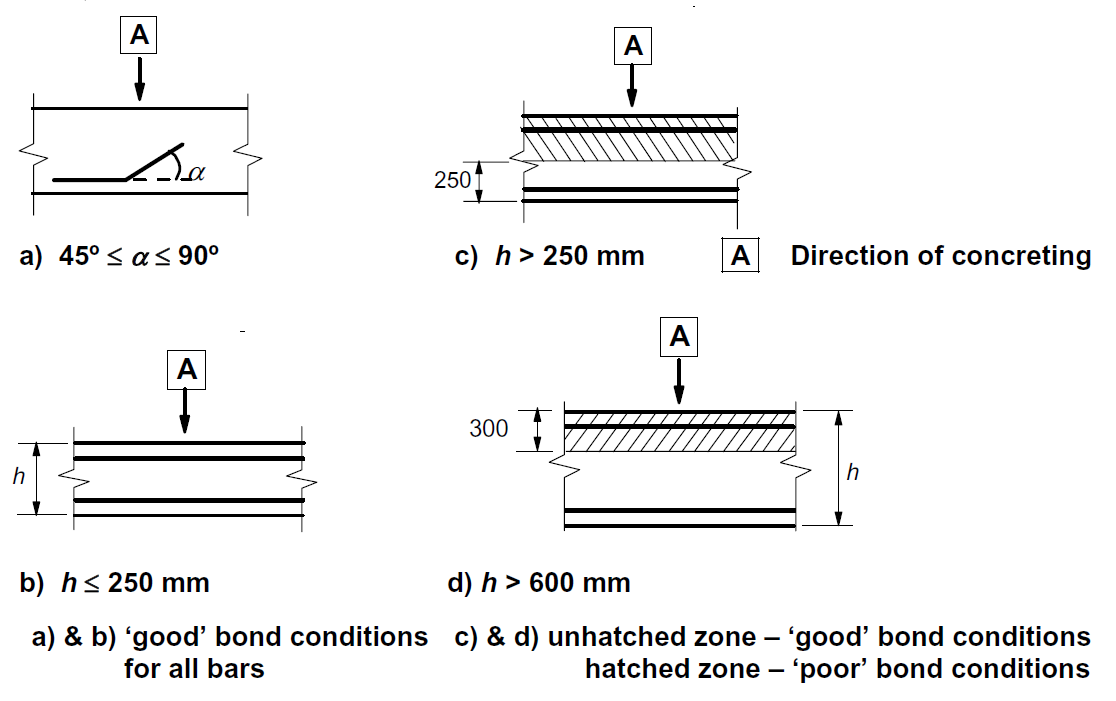### Choose language# Ultimate limit state analysis

$$The different verifications required by EN 1992-1-1 are assessed based on the direct results provided by the model. ULS verifications are carried out for concrete strength, reinforcement strength, and anchorage (bond shear stresses).The concrete strength in compression is evaluated as the ratio between the maximum principal compressive stress σc3 obtained from FE analysis and the limit value σc3,lim. Then:$\frac{σ_{c3}}{σ_{c3,lim}}$$σ_{c3,lim} = f_{cd} = α_{cc} \cdot \frac{f_{ck,red}}{γ_c} = α_{cc} \cdot \frac{k_c \cdot f_{ck}}{γ_c} = α_{cc} \cdot \frac{\eta _{fc} \cdot k_{c2} \cdot f_{ck}}{γ_c}$${\eta _{fc}} = {\left( {\frac{{30}}{{{f_{ck}}}}} \right)^{\frac{1}{3}}} \le 1$where:fck characteristic cylinder strength of concrete,kc2 compression softening factor (see 5.1.1),γc is the partial safety factor for concrete, γc = 1,5,αcc is the coefficient taking account of long term effects on the compressive strength and of unfavourable effects resulting from the way the load is applied. The default value is 1,0. The strength of the reinforcement is evaluated in both tension and compression as the ratio between the stress in the reinforcement at the cracks σsr and the specified limit value σsr,lim:$\frac{σ_{sr}}{σ_{sr,lim}}$$$σ_{c3,lim} = \frac{k \cdot f_{yk}}{γ_s}\qquad\qquad\textsf{\small{for bilinear diagram with inclined top branch}}$$$$σ_{c3,lim} = \frac{f_{yk}}{γ_s}\qquad\qquad\,\,\,\,\textsf{\small{for bilinear diagram with horizontal top branch}}$$where:fyk yield strength of the reinforcement,k the ratio of tensile strength ftk to the yield stress, $$k = \frac{f_{tk}}{f_{yk}}$$γs is the partial safety factor for reinforcement γs = 1,15.The bond shear stress is evaluated independently as the ratio between the bond stress τb calculated by FE analysis and the ultimate bond strength fbd, according to EN 1992-1-1 chap. 8.4.2:$\frac{τ_{b}}{f_{bd}}$$f_{bd} = 2.25 \cdot η_1\cdot η_2\cdot f_{ctd}$where: fctd is the design value of concrete tensile strength. Due to the increasing brittleness of higher strength concrete, fctk,0,05 is limited to the value for C60/75, η1 is a coefficient related to the quality of the bond condition and the position of the bar during concreting (Fig. 33): η1 = 1,0 when ‘good’ conditions are obtained and η1 = 0,7 for all other cases and for bars in structural elements built with slip-forms, unless it can be shown that ‘good’ bond conditions existη2 is related to the bar diameter: η2 = 1,0 for Ø ≤ 32 mm η2 = (132 - Ø)/100 for Ø > 32 mm$\textsf{\textit{\footnotesize{Fig. 33\qquad Description of bond conditions.}}}$These verifications are carried out with respect to the appropriate limit values for the respective parts of the structure (i.e., in spite of having a single grade both for concrete and reinforcement material, the final stress-strain diagrams will differ in each part of the structure due to tension stiffening and compression softening effects).$$